#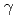ray measuring method for a simple determination of radioactivity of uranium in waste drums
For determining radioactive of waste drums using passiveray, we showed a new analysis method deriving the linear relation between radiation source distribution andray count rate under the homogeneous waste density. On the other hand, there were problem applying the linear relation to the case of inhomogeneous waste density. We proposed to measure theray count rate at the positions on spherical surface surrounding the waste drum. Intending to create the approximative homogeneous waste density state, we proposed to calculate the geometric mean of measurements. As a result, we showed a relation under the inhomogeneous waste density. The relation is represented by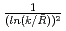and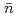, where "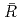" is the geometric mean of tworay (766 keV and 1001 keV) count rates ratios. "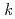" is the ratio of emission rates of the tworays. "" are the geometric mean ofray (1001 keV) count rates.